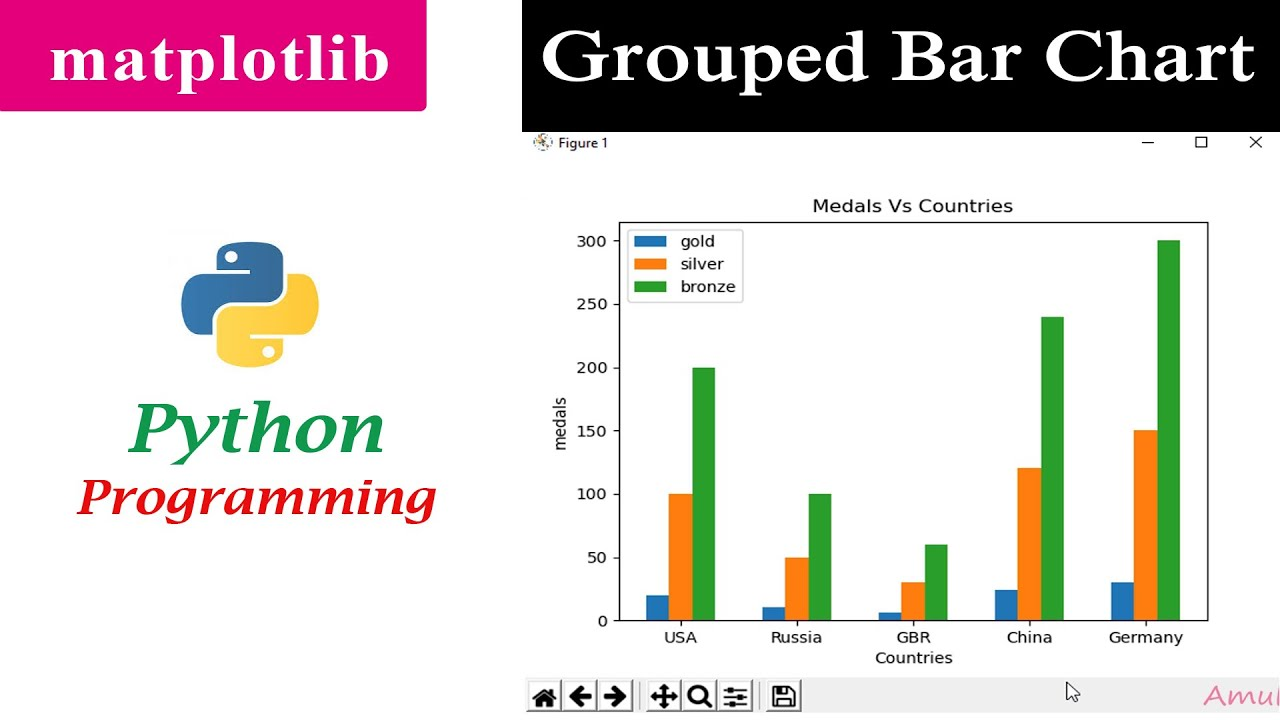# Multiple Bar Chart | Grouped Bar Graph | MatplotlibIn this Python Programming video tutorial you will learn about multiple bar chart or grouped bar graph in matplotlib in detail.

In this Python Programming video tutorial you will learn about multiple bar chart or grouped bar graph in matplotlib in detail.

Matplotlib is a plotting library for the Python programming language and its numerical mathematics extension NumPy. You can represent the data in different types of plot. Line Plot Scatter plot Histograms Bar graph Pie Chart Area Plot

In the bar chart you can place the different bar of same category next to each other and can form multiple bar chart.

## Matplotlib Tutorial | Python Matplotlib Library

Matplotlib Tutorial. Matplotlib is one of the most popular Python packages used for data visualization. It is a cross-platform library for making 2D plots from data in arrays. It provides an object-oriented API that helps in embedding plots in applications using Python GUI toolkits such as PyQt, WxPythonotTkinter.

## Python Matplotlib Introduction & Installation

This is our first tutorial in Python Matplotlib, in this tutorial we are going to learn about Python Matplotlib Introduction & Installation.

## Python Matplotlib How to Plot Points

In this article we are going to learn Matplotlib How to Plot Points. also we can call it scatter plot.  you

## Python Matplotlib Plotting Barchart - Code Loop

A vertical bar chart is sometimes called a column chart. you can read the previous articles for Python Matplotlib in the below links.

## Python Tricks Every Developer Should Know

In this tutorial, you’re going to learn a variety of Python tricks that you can use to write your Python code in a more readable and efficient way like a pro.